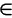# Maths - Field

note: The concept of a 'field' in algebra is different from the concept of a 'vector or scalar field' which occurs in geometry and physics. (although there is a connection with vectors as we shall see).

### Definitions

A field is a commutative ring with unity.

An integral domain is a commutative ring with unity and no zero-divisors.

A zero-divisor is a non-zero element 'a' of a commutative ring 'R' such that there is a non-zero element bR with ab=0

### Axioms

associativity (a+b)+c=a+(b+c) (a*b)*c=a*(b*c)
commutativity a+b=b+a a*b=b*a
distributivity     a*(b+c)=a*b+a*c
(a+b)*c=a*c+b*c
identity a+0 = a
0+a = a
a*1 = a
1*a = a

inverses a+(-a) = 0
(-a)+a = 0
a*a-1 = 1
a-1*a = 1
if a≠0

### Examples of Fields

Examples include:

• the real number field.
• the complex numbers C.
• the rational numbers Q.
• finite fields.
• various fields of functions.

Some algebras that are not fields are vectors (unless a commutative multiplication is used), matrices (not commutative) and quaternions (not commutative).

### Properties

Every field is an integral domain

### Extension Field

A Field 'E' is an extension field of a field 'F' if F is a subset of E and the operations of F are those of E restricted to F.

### Polynomial Domain

Every algebraic number field can be obtained as the quotient of the polynomial domain Q[x] by the principle ideal generated by an irreducible polynomial.

### Theorems

The degree of an extension Q(r) always matches the degree of the irreducible polynomial to which r is a root.

The degree of a normal extension matches the degree of its Galois group.

p(x)

F

Field

E

Extension

splitting of p(x)
x²-2 Q Q(√2) (x-√2)(x+√2)
Q[z]/<z²-2> (x-z)(x+z)
x³-2 Q Q(w,³√2) (x-³√2)(x-w ³√2)(x-w² ³√2)
Q(w)[z]/<z³-2> (x-z)(x-w z)(x-w² z)
Q(³√2)[y]/<y² + y + 1> (x-³√2)(x-y ³√2)(x-y² ³√2)

Where I can, I have put links to Amazon for books that are relevant to the subject, click on the appropriate country flag to get more details of the book or to buy it from them.The Princeton Companion to Mathematics - This is a big book that attempts to give a wide overview of the whole of mathematics, inevitably there are many things missing, but it gives a good insight into the history, concepts, branches, theorems and wider perspective of mathematics. It is well written and, if you are interested in maths, this is the type of book where you can open a page at random and find something interesting to read. To some extent it can be used as a reference book, although it doesn't have tables of formula for trig functions and so on, but where it is most useful is when you want to read about various topics to find out which topics are interesting and relevant to you.